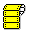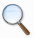<-- Home

##Words in category Arithmetic in glossary Certified Forth WordsThe following words are listed in the category Arithmetic (8 words found):

 Word Name Word Type Data Stack Signature Return Stack Signature Description 200x Ref Availability 200x ANS F83 FIG - S n1 | u1 n2 | u2 -- n3 | u3 -- Subtract n2 | u2 from n1 | u1, giving the difference n3 | u3. 6.1.0160 * S n1 | u1 n2 | u2 -- n3 | u3 -- Multiply n1 | u1 by n2 | u2 giving the product n3 | u3. 6.1.0090 */MOD S n1 n2 n3 -- n4 n5 -- Multiply n1 by n2 producing the intermediate double-cell result d. Divide d by n3 producing the single-cell remainder n4 and the single-cell quotient n5. An ambiguous condition exists if n3 is zero, or if the quotient n5 lies outside the range of a single-cell signed integer. If d and n3 differ in sign, the implementation-defined result returned will be the same as that returned by either the phrase >R M* R> FM/MOD or the phrase >R M* R> SM/REM. 6.1.0110 / S n1 n2 -- n3 -- Divide n1 by n2, giving the single-cell quotient n3. An ambiguous condition exists if n2 is zero. If n1 and n2 differ in sign, the implementation-defined result returned will be the same as that returned by either the phrase >R S>D R> FM/MOD SWAP DROP or the phrase >R S>D R> SM/REM SWAP DROP. 6.1.0230 + S n1 | u1 n2 | u2 -- n3 | u3 -- Add n2 | u2 to n1 | u1, giving the sum n3 | u3. 6.1.0120 2* S n1 -- n2 -- x2 is the result of shifting x1 one bit toward the most-significant bit, filling the vacated least-significant bit with zero. 6.1.0320 2/ S x1 -- x2 -- x2 is the result of shifting x1 one bit toward the least-significant bit, leaving the most-significant bit unchanged. 6.1.0330 MOD S n1 n2 -- n3 -- Divide n1 by n2, giving the single-cell remainder n3. An ambiguous condition exists if n2 is zero. If n1 and n2 differ in sign, the implementation-defined result returned will be the same as that returned by either the phrase >R S>D R> FM/MOD DROP or the phrase >R S>D R> SM/REM DROP. 6.1.1890